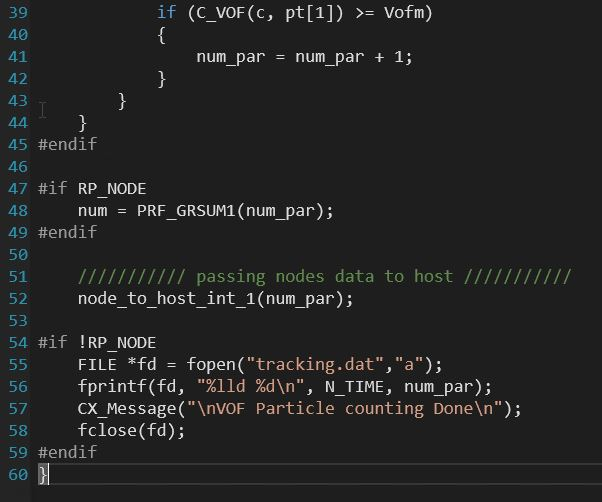# FLUENT VOF + DPM with UDF looping all particles in parallel model

Hi all,

I am working on a VOF+DPM model, in which I would like to calculate how many particles are in the 2nd phase after each flow time step. The UDF seems to work in serial mode, but in parallel mode, the calculation is not accurate due to the unknown issue with the UDF.

When in parallel mode, at the end of each time step, the UDF loops all the particles to verify the volume fraction of the 2nd phase in the cell which contains the particle is larger than a specific value (Vofm), and then writes down the number of particles to a local file. However, as the parallel processes increase, the total number of particles in 2nd phase increases at the same time step.

I am using hybrid method for DPM model and below is the UDF.

Any help is appreciated!

•  #include "udf.h"

#include "dpm.h"

#include "math.h"

#define Vofm 1.0   // define vof_m volume to judge the particle to be counted or not

int num_par; // number of particles in phase 2

DEFINE_EXECUTE_AT_END(execute_at_end)

{

#if !RP_NODE

num_par = 0;

#endif

#if !RP_HOST

num_par = 0;

int zone_ID=63;           // subdomain, make sure different domains have diferent IDs

Domain *d=Get_Domain(1);

• Particle *p;

Injection *I;

Injection *dpm_injections = Get_dpm_injections();

// iterate all injections and particles

loop(I,dpm_injections)

{

loop(p,I->p_init)

{

cell_t c;

c=P_CELL(p);

if (C_VOF(c, pt) >= Vofm)

{

num_par = num_par + 1;

}

}

}

#endif

•Apologize for posting too many posts as someshow the access was denied when I tried to post everything together.

• edited June 26

Hard to comment: what is now wrong? Can you debug the parallel part line by line?

• Hi Amine, for example, I tested the UDF by setting Vofm to 1.0 (if volume fraction of 2nd phase is higher or equal to Vofm, then number of particle +1), and ran it with 16 processes. The number of particles in the celll with Vofm >= 1.0 was zero. However, when I changed the processes to 64, the number of particles was larger than zero.

• edited June 26

Can you debug the parallel part line by line? as I said hard to comment here especially as you splitted the post into 3 parts. Just to let you know that the default behavior is that particles are only tracked with primary phase.

I think you have some issues with your parallelization of the UDF.